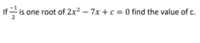# Factoring / Partial Factoring Of A Quadratic Equation

#### approcotE

##### New memberHey, I'm in Grade 11 Functions and I've got a question that I'm kinda stuck on. The question is above. I'm pretty sure you need to partially factor this equation but after that, I'm honestly not sure what to do.

Here's what I tried out so far (don't bully me): So 2x^2-7x+c can be partially factored to get x(2x-7)+c=0. The roots here are like x=0 and x=7/2. Do I need to substitute -1/2 for one of the roots, or do I need to like substitute one x value with -1/2, or?

That's all I know, If anyone could help me out?#### Harry_the_cat

##### Senior Member
I'll walk you through some steps, but you have to answer my questions. There are several ways to do this.
If a number is a root of an equation, what does that really mean?

#### Dr.Peterson

##### Elite Member
View attachment 22325

Hey, I'm in Grade 11 Functions and I've got a question that I'm kinda stuck on. The question is above. I'm pretty sure you need to partially factor this equation but after that, I'm honestly not sure what to do.

Here's what I tried out so far (don't bully me): So 2x^2-7x+c can be partially factored to get x(2x-7)+c=0. The roots here are like x=0 and x=7/2. Do I need to substitute -1/2 for one of the roots, or do I need to like substitute one x value with -1/2, or?

That's all I know, If anyone could help me out?"Partial factoring" like this doesn't accomplish anything toward solving the equation. It is not true that x=0 and x=7/2 are roots of x(2x-7)+c=0; they certainly don't make it true unless c = 0.

What should happen if you replace x with -1/2 in the given (original) equation?

#### JeffM

##### Elite Member
As the resident genius feline implied, if -1/2 is a root of that equation, then

$$\displaystyle 2\left ( - \dfrac{1}{2} \right )^2 - 7 \left (-\dfrac{1}{2} \right ) + c = 0.$$

Can you solve for c?

The question merely involves knowing what a function is and what its roots mean.

•Jomo and topsquark

#### Otis

##### Elite Member
… do I need to … substitute one x value with -1/2 …
Hi approcotE. You need to substitute -1/2 for each x, not just one.

The question may be reworded:

If x = -1/2 is a solution to the equation

2x2 - 7x + c = 0

then find the value of c.

In order to solve for c, we need an equation in which c is the only unknown present. Setting x=-1/2 gives us such an equation.Last edited:

#### Otis

##### Elite Member
… [it] involves knowing what a function is …
Hi Jeff. Introducing the concept of function before assigning such exercises could be ideal, but I don't think it's necessary.

I'm wondering how the course defined 'root', as well as whether the OP rephrased the given exercise statement.#### JeffM

##### Elite Member
Hi Jeff. Introducing the concept of function before assigning such exercises could be ideal, but I don't think it's necessary.

I'm wondering how the course defined 'root', as well as whether the OP rephrased the given exercise statement.Hey there Otis.

The OP said "I'm in Grade 11 Functions." (The OP seems to have actually read our guidelines intelligently.) The idea that solving an equation is the same as finding the root of a function is one that should be hit on emphatically early in such a course. Too many courses seem to treat functions as just preparation for calculus.

#### Otis

##### Elite Member
… The OP seems to have actually read our guidelines …
Indeed.

My comment about 'function' was meant to be in general. I've seen the wording in the op before (where 'root' just means 'solution', when referring to an equation). Students would've been taught enough to know that the given solution -1/2 is the independent variable's value, so they make the substitution and solve for c. Introduction to functions could happen one or two quarters later.